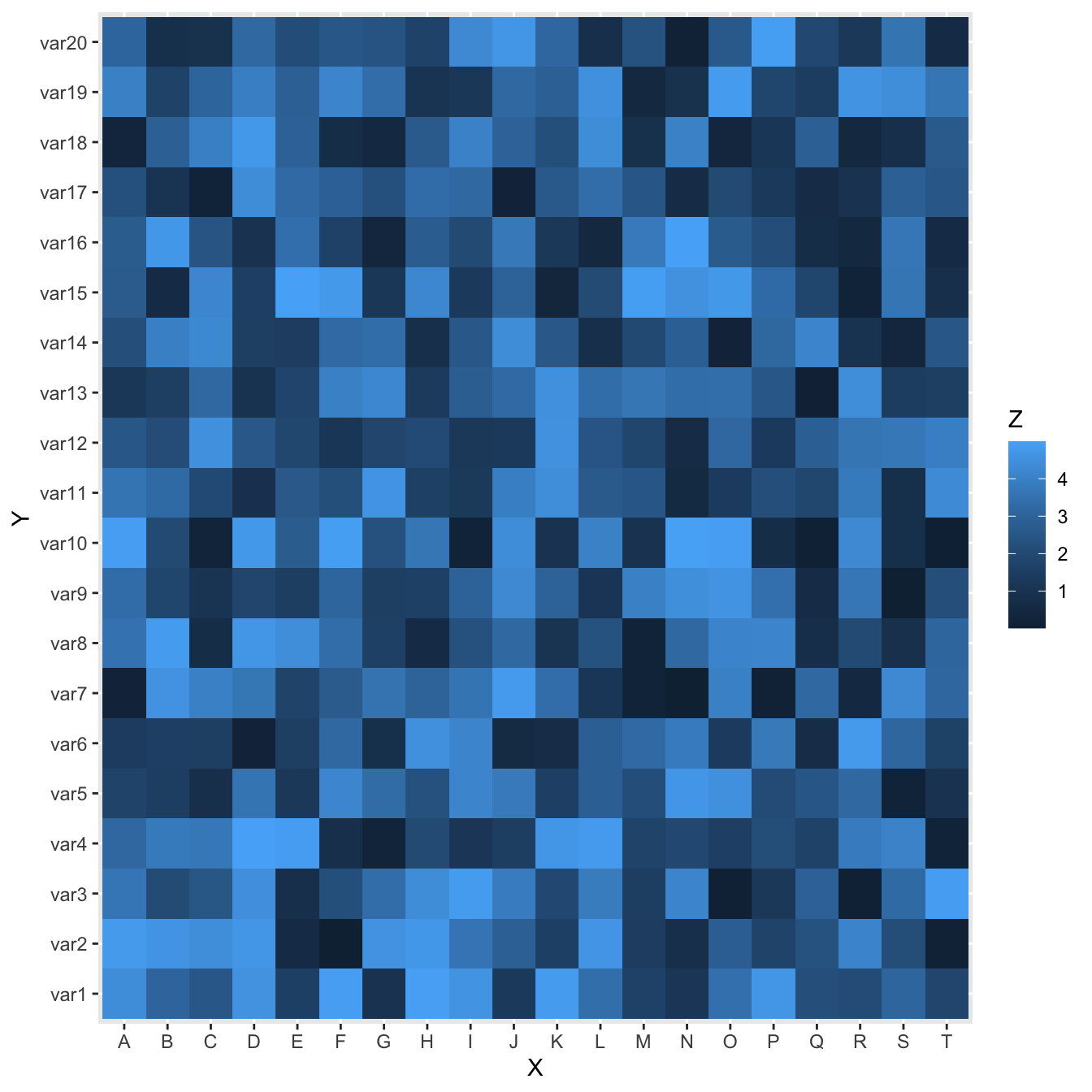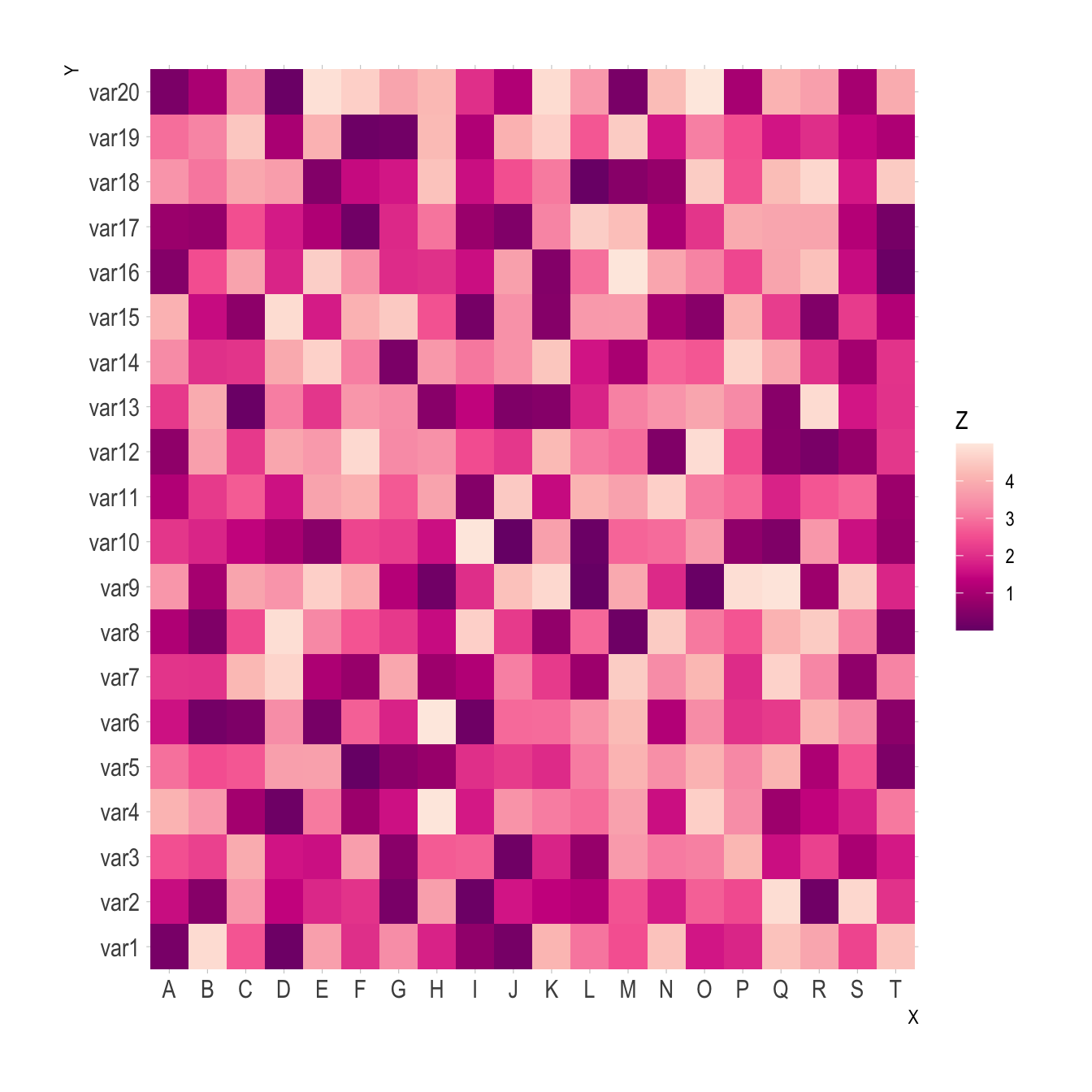# ggplot2 heatmap

This document provides several examples of heatmaps built with `R` and ggplot2. It describes the main customization you can apply, with explanation and reproducible code.
Note: The native heatmap() function provides more options for data normalization and clustering. Consider it as a valuable option.

# Most basic heatmap with `ggplot2`

This is the most basic heatmap you can build with `R` and `ggplot2`, using the `geom_tile()` function.

Input data must be a long format where each row provides an observation. At least 3 variables are needed per observation:

• `x`: position on the X axis
• `y`: position on the Y axis
• `fill`: the numeric value that will be translated in a color``````# Library
library(ggplot2)

# Dummy data
x <- LETTERS[1:20]
y <- paste0("var", seq(1,20))
data <- expand.grid(X=x, Y=y)
data\$Z <- runif(400, 0, 5)

# Heatmap
ggplot(data, aes(X, Y, fill= Z)) +
geom_tile()``````

# Control color paletteColor palette can be changed like in any ggplot2 chart. Above are 3 examples using different methods:

• `scale_fill_gradient()` to provide extreme colors of the palette
• `scale_fill_distiller)` to provide a ColorBrewer palette
• `scale_fill_viridis()` to use Viridis. Do not forget `discrete=FALSE` for a continuous variable.
``````# Library
library(ggplot2)
library(hrbrthemes)

# Dummy data
x <- LETTERS[1:20]
y <- paste0("var", seq(1,20))
data <- expand.grid(X=x, Y=y)
data\$Z <- runif(400, 0, 5)

# Give extreme colors:
ggplot(data, aes(X, Y, fill= Z)) +
geom_tile() +
scale_fill_gradient(low="white", high="blue") +
theme_ipsum()

# Color Brewer palette
ggplot(data, aes(X, Y, fill= Z)) +
geom_tile() +
scale_fill_distiller(palette = "RdPu") +
theme_ipsum()

# Color Brewer palette
library(viridis)
ggplot(data, aes(X, Y, fill= Z)) +
geom_tile() +
scale_fill_viridis(discrete=FALSE) +
theme_ipsum()``````

# From wide input format

It is a common issue to have a wide matrix as input, as for the `volcano` dataset. In this case, you need to tidy it with the `gather()` function of the `tidyr` package to visualize it with ggplot.``````# Library
library(ggplot2)
library(tidyr)
library(tibble)
library(hrbrthemes)
library(dplyr)

# Volcano dataset
#volcano

# Heatmap
volcano %>%

# Data wrangling
as_tibble() %>%
rowid_to_column(var="X") %>%
gather(key="Y", value="Z", -1) %>%

# Change Y to numeric
mutate(Y=as.numeric(gsub("V","",Y))) %>%

# Viz
ggplot(aes(X, Y, fill= Z)) +
geom_tile() +
theme_ipsum() +
theme(legend.position="none")``````

# Turn it interactive with `plotly`

One of the nice feature of ggplot2 is that charts can be turned interactive in seconds thanks to `plotly`. You just need to wrap your chart in an object and call it in the `ggplotly()` function.

Often, it is a good practice to custom the text available in the tooltip.

Note: try to hover cells to see the tooltip, select an area to zoom in.

``````# Library
library(ggplot2)
library(hrbrthemes)
library(plotly)

# Dummy data
x <- LETTERS[1:20]
y <- paste0("var", seq(1,20))
data <- expand.grid(X=x, Y=y)
data\$Z <- runif(400, 0, 5)

# new column: text for tooltip:
data <- data %>%
mutate(text = paste0("x: ", x, "\n", "y: ", y, "\n", "Value: ",round(Z,2), "\n", "What else?"))

# classic ggplot, with text in aes
p <- ggplot(data, aes(X, Y, fill= Z, text=text)) +
geom_tile() +
theme_ipsum()

ggplotly(p, tooltip="text")

# save the widget
# library(htmlwidgets)
# saveWidget(pp, file=paste0( getwd(), "/HtmlWidget/ggplotlyHeatmap.html"))``````

Related chart types

## Contact

This document is a work by Yan Holtz. Any feedback is highly encouraged. You can fill an issue on Github, drop me a message on Twitter, or send an email pasting yan.holtz.data with gmail.com.